### C Ya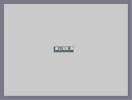Hover over the thumbnail for a full-size version.

Author RavenMac author:ravenmac n-art nonplayable unrated 2008-07-11 2008-07-11 \$C Ya#RavenMac#none#00000000000000000000000000000000000000000000000000000000000000000000000000000000000000000000000000000000000000000000000000000000000000000000000000000000000000000000000000000000000000000000000000000000000000000000000000000000000000000000000000000000000000000000000000000000000000000000000000000000000000000000000000000000000000000000000000000000000000000000000000000000000000000000000000000000000000000000000000000000000000000000000000000000000000000000000000000000000000000000000000000000000000000000000000000000000000000000000000000000000000000000000000000000000000000000000000000000000000000000000000000000000000000000000000000000000000000000000000000000000000000000000000000000000000000000000000000000000000000|1^332,304!1^333,304!1^334,304!1^335,304!1^336,304!1^337,304!1^338,304!1^339,304!1^340,304!1^341,304!1^342,304!1^343,304!1^344,304!1^345,304!1^346,304!1^347,304!1^348,304!1^349,304!1^350,304!1^351,304!1^352,304!1^353,304!1^354,304!1^355,304!1^356,304!1^357,304!1^358,304!1^359,304!1^360,304!1^361,304!1^362,304!1^363,304!1^364,304!1^365,304!1^366,304!1^367,304!1^368,304!1^369,304!1^370,304!1^371,304!1^372,304!1^373,304!1^374,304!1^375,304!1^376,304!1^377,304!1^378,304!1^379,304!1^380,304!1^381,304!1^382,304!1^383,304!1^384,304!1^385,304!1^386,304!1^387,304!1^388,304!1^389,304!1^390,304!1^391,304!1^392,304!1^393,304!1^394,304!1^395,304!1^396,304!1^397,304!1^398,304!1^399,304!1^400,304!1^401,304!1^402,304!1^403,304!1^404,304!1^405,304!1^406,304!1^407,304!1^408,304!1^409,304!1^410,304!1^411,304!1^412,304!1^413,304!1^414,304!1^415,304!1^416,304!1^417,304!1^418,304!1^419,304!1^420,304!1^421,304!1^422,304!1^423,304!1^424,304!1^425,304!1^426,304!1^427,304!1^428,304!1^429,304!1^430,304!1^431,304!1^432,304!1^332,303!1^333,303!1^334,303!1^335,303!1^336,303!1^337,303!1^338,303!1^339,303!1^340,303!1^341,303!1^342,303!1^343,303!1^344,303!1^345,303!1^346,303!1^347,303!1^348,303!1^349,303!1^350,303!1^351,303!1^352,303!1^353,303!1^354,303!1^355,303!1^356,303!1^357,303!1^358,303!1^359,303!1^360,303!1^361,303!1^362,303!1^363,303!1^364,303!1^365,303!1^366,303!1^367,303!1^368,303!1^369,303!1^370,303!1^371,303!1^372,303!1^373,303!1^374,303!1^375,303!1^376,303!1^377,303!1^378,303!1^379,303!1^380,303!1^381,303!1^382,303!1^383,303!1^384,303!1^385,303!1^386,303!1^387,303!1^388,303!1^389,303!1^390,303!1^391,303!1^392,303!1^393,303!1^394,303!1^395,303!1^396,303!1^397,303!1^398,303!1^399,303!1^400,303!1^401,303!1^402,303!1^403,303!1^404,303!1^405,303!1^406,303!1^407,303!1^408,303!1^409,303!1^410,303!1^411,303!1^412,303!1^413,303!1^414,303!1^415,303!1^416,303!1^417,303!1^418,303!1^419,303!1^420,303!1^421,303!1^422,303!1^423,303!1^424,303!1^425,303!1^426,303!1^427,303!1^428,303!1^429,303!1^430,303!1^431,303!1^432,303!1^332,302!1^333,302!1^334,302!1^335,302!1^336,302!1^337,302!1^338,302!1^339,302!1^340,302!1^341,302!1^342,302!1^343,302!1^344,302!1^345,302!1^346,302!1^347,302!1^348,302!1^349,302!1^350,302!1^351,302!1^352,302!1^353,302!1^354,302!1^355,302!1^356,302!1^357,302!1^358,302!1^359,302!1^360,302!1^361,302!1^362,302!1^363,302!1^364,302!1^365,302!1^366,302!1^367,302!1^368,302!1^369,302!1^370,302!1^371,302!1^372,302!1^373,302!1^374,302!1^375,302!1^376,302!1^377,302!1^378,302!1^379,302!1^380,302!1^381,302!1^382,302!1^383,302!1^384,302!1^385,302!1^386,302!1^387,302!1^388,302!1^389,302!1^390,302!1^391,302!1^392,302!1^393,302!1^394,302!1^395,302!1^396,302!1^397,302!1^398,302!1^399,302!1^400,302!1^401,302!1^402,302!1^403,302!1^404,302!1^405,302!1^406,302!1^407,302!1^408,302!1^409,302!1^410,302!1^411,302!1^412,302!1^413,302!1^414,302!1^415,302!1^416,302!1^417,302!1^418,302!1^419,302!1^420,302!1^421,302!1^422,302!1^423,302!1^424,302!1^425,302!1^426,302!1^427,302!1^428,302!1^429,302!1^430,302!1^431,302!1^432,302!1^332,301!1^333,301!1^334,301!1^335,301!1^336,301!1^337,301!1^338,301!1^339,301!1^340,301!1^341,301!1^342,301!1^343,301!1^344,301!1^345,301!1^346,301!1^347,301!1^348,301!1^349,301!1^350,301!1^351,301!1^352,301!1^353,301!1^354,301!1^355,301!1^356,301!1^357,301!1^358,301!1^359,301!1^360,301!1^361,301!1^362,301!1^363,301!1^364,301!1^365,301!1^366,301!1^367,301!1^368,301!1^369,301!1^370,301!1^371,301!1^372,301!1^373,301!1^374,301!1^375,301!1^376,301!1^377,301!1^378,301!1^379,301!1^380,301!1^381,301!1^382,301!1^383,301!1^384,301!1^385,301!1^386,301!1^387,301!1^388,301!1^389,301!1^390,301!1^391,301!1^392,301!1^393,301!1^394,301!1^395,301!1^396,301!1^397,301!1^398,301!1^399,301!1^400,301!1^401,301!1^402,301!1^403,301!1^404,301!1^405,301!1^406,301!1^407,301!1^408,301!1^409,301!1^410,301!1^411,301!1^412,301!1^413,301!1^414,301!1^415,301!1^416,301!1^417,301!1^418,301!1^419,301!1^420,301!1^421,301!1^422,301!1^423,301!1^424,301!1^425,301!1^426,301!1^427,301!1^428,301!1^429,301!1^430,301!1^431,301!1^432,301!1^332,300!1^333,300!1^334,300!1^335,300!1^336,300!1^337,300!1^338,300!1^339,300!1^340,300!1^341,300!1^342,300!1^343,300!1^344,300!1^345,300!1^346,300!1^347,300!1^348,300!1^349,300!1^350,300!1^351,300!1^352,300!1^353,300!1^354,300!1^355,300!1^356,300!1^357,300!1^358,300!1^359,300!1^360,300!1^361,300!1^362,300!1^363,300!1^364,300!1^365,300!1^366,300!1^367,300!1^368,300!1^369,300!1^370,300!1^371,300!1^372,300!1^373,300!1^374,300!1^375,300!1^376,300!1^377,300!1^378,300!1^379,300!1^380,300!1^381,300!1^382,300!1^383,300!1^384,300!1^385,300!1^386,300!1^387,300!1^388,300!1^389,300!1^390,300!1^391,300!1^392,300!1^393,300!1^394,300!1^395,300!1^396,300!1^397,300!1^398,300!1^399,300!1^400,300!1^401,300!1^402,300!1^403,300!1^404,300!1^405,300!1^406,300!1^407,300!1^408,300!1^409,300!1^410,300!1^411,300!1^412,300!1^413,300!1^414,300!1^415,300!1^416,300!1^417,300!1^418,300!1^419,300!1^420,300!1^421,300!1^422,300!1^423,300!1^424,300!1^425,300!1^426,300!1^427,300!1^428,300!1^429,300!1^430,300!1^431,300!1^432,300!1^332,299!1^333,299!1^334,299!1^335,299!1^336,299!1^337,299!1^338,299!1^339,299!1^340,299!1^341,299!1^342,299!1^343,299!1^344,299!1^345,299!1^346,299!1^347,299!1^348,299!1^349,299!1^350,299!1^351,299!1^352,299!1^353,299!1^354,299!1^355,299!1^356,299!1^357,299!1^358,299!1^359,299!1^360,299!1^361,299!1^362,299!1^363,299!1^364,299!1^365,299!1^366,299!1^367,299!1^368,299!1^369,299!1^370,299!1^371,299!1^372,299!1^373,299!1^374,299!1^375,299!1^376,299!1^377,299!1^378,299!1^379,299!1^380,299!1^381,299!1^382,299!1^383,299!1^384,299!1^385,299!1^386,299!1^387,299!1^388,299!1^389,299!1^390,299!1^391,299!1^392,299!1^393,299!1^394,299!1^395,299!1^396,299!1^397,299!1^398,299!1^399,299!1^400,299!1^401,299!1^402,299!1^403,299!1^404,299!1^405,299!1^406,299!1^407,299!1^408,299!1^409,299!1^410,299!1^411,299!1^412,299!1^413,299!1^414,299!1^415,299!1^416,299!1^417,299!1^418,299!1^419,299!1^420,299!1^421,299!1^422,299!1^423,299!1^424,299!1^425,299!1^426,299!1^427,299!1^428,299!1^429,299!1^430,299!1^431,299!1^432,299!1^332,298!1^333,298!1^334,298!1^335,298!1^336,298!1^337,298!1^338,298!1^339,298!1^340,298!1^341,298!1^342,298!1^343,298!1^344,298!1^345,298!1^346,298!1^347,298!1^348,298!1^349,298!1^350,298!1^351,298!1^352,298!1^353,298!1^354,298!1^355,298!1^356,298!1^357,298!1^358,298!1^359,298!1^360,298!1^361,298!1^362,298!1^363,298!1^364,298!1^365,298!1^366,298!1^367,298!1^368,298!1^369,298!1^370,298!1^371,298!1^372,298!1^373,298!1^374,298!1^375,298!1^376,298!1^377,298!1^378,298!1^379,298!1^380,298!1^381,298!1^382,298!1^383,298!1^384,298!1^385,298!1^386,298!1^387,298!1^388,298!1^389,298!1^390,298!1^391,298!1^392,298!1^393,298!1^394,298!1^395,298!1^396,298!1^397,298!1^398,298!1^399,298!1^400,298!1^401,298!1^402,298!1^403,298!1^404,298!1^405,298!1^406,298!1^407,298!1^408,298!1^409,298!1^410,298!1^411,298!1^412,298!1^413,298!1^414,298!1^415,298!1^416,298!1^417,298!1^418,298!1^419,298!1^420,298!1^421,298!1^422,298!1^423,298!1^424,298!1^425,298!1^426,298!1^427,298!1^428,298!1^429,298!1^430,298!1^431,298!1^432,298!1^332,297!1^333,297!1^334,297!1^335,297!1^336,297!1^337,297!1^338,297!1^339,297!1^340,297!1^341,297!1^342,297!1^343,297!1^344,297!1^345,297!1^346,297!1^347,297!1^348,297!1^349,297!1^350,297!1^351,297!1^352,297!1^353,297!1^354,297!1^355,297!1^356,297!1^357,297!1^358,297!1^359,297!1^360,297!1^361,297!1^362,297!1^363,297!1^364,297!1^365,297!1^366,297!1^367,297!1^368,297!1^369,297!1^370,297!1^371,297!1^372,297!1^373,297!1^374,297!1^375,297!1^376,297!1^377,297!1^378,297!1^379,297!1^380,297!1^381,297!1^382,297!1^383,297!1^384,297!1^385,297!1^386,297!1^387,297!1^388,297!1^389,297!1^390,297!1^391,297!1^392,297!1^393,297!1^394,297!1^395,297!1^396,297!1^397,297!1^398,297!1^399,297!1^400,297!1^401,297!1^402,297!1^403,297!1^404,297!1^405,297!1^406,297!1^407,297!1^408,297!1^409,297!1^410,297!1^411,297!1^412,297!1^413,297!1^414,297!1^415,297!1^416,297!1^417,297!1^418,297!1^419,297!1^420,297!1^421,297!1^422,297!1^423,297!1^424,297!1^425,297!1^426,297!1^427,297!1^428,297!1^429,297!1^430,297!1^431,297!1^432,297!1^332,296!1^333,296!1^334,296!1^335,296!1^336,296!1^337,296!1^338,296!1^339,296!1^340,296!1^341,296!1^342,296!1^343,296!1^344,296!1^345,296!1^346,296!1^347,296!1^348,296!1^349,296!1^350,296!1^351,296!1^352,296!1^353,296!1^354,296!1^355,296!1^356,296!1^357,296!1^358,296!1^359,296!1^360,296!1^361,296!1^362,296!1^363,296!1^364,296!1^365,296!1^366,296!1^367,296!1^368,296!1^369,296!1^370,296!1^371,296!1^372,296!1^373,296!1^374,296!1^375,296!1^376,296!1^377,296!1^378,296!1^379,296!1^380,296!1^381,296!1^382,296!1^383,296!1^384,296!1^385,296!1^386,296!1^387,296!1^388,296!1^389,296!1^390,296!1^391,296!1^392,296!1^393,296!1^394,296!1^395,296!1^396,296!1^397,296!1^398,296!1^399,296!1^400,296!1^401,296!1^402,296!1^403,296!1^404,296!1^405,296!1^406,296!1^407,296!1^408,296!1^409,296!1^410,296!1^411,296!1^412,296!1^413,296!1^414,296!1^415,296!1^416,296!1^417,296!1^418,296!1^419,296!1^420,296!1^421,296!1^422,296!1^423,296!1^424,296!1^425,296!1^426,296!1^427,296!1^428,296!1^429,296!1^430,296!1^431,296!1^432,296!1^332,295!1^333,295!1^334,295!1^335,295!1^336,295!1^337,295!1^338,295!1^339,295!1^340,295!1^341,295!1^342,295!1^343,295!1^344,295!1^345,295!1^346,295!1^347,295!1^348,295!1^349,295!1^350,295!1^351,295!1^352,295!1^353,295!1^354,295!1^355,295!1^356,295!1^357,295!1^358,295!1^359,295!1^360,295!1^361,295!1^362,295!1^363,295!1^364,295!1^365,295!1^366,295!1^367,295!1^368,295!1^369,295!1^370,295!1^371,295!1^372,295!1^373,295!1^374,295!1^375,295!1^376,295!1^377,295!1^378,295!1^379,295!1^380,295!1^381,295!1^382,295!1^383,295!1^384,295!1^385,295!1^386,295!1^387,295!1^388,295!1^389,295!1^390,295!1^391,295!1^392,295!1^393,295!1^394,295!1^395,295!1^396,295!1^397,295!1^398,295!1^399,295!1^400,295!1^401,295!1^402,295!1^403,295!1^404,295!1^405,295!1^406,295!1^407,295!1^408,295!1^409,295!1^410,295!1^411,295!1^412,295!1^413,295!1^414,295!1^415,295!1^416,295!1^417,295!1^418,295!1^419,295!1^420,295!1^421,295!1^422,295!1^423,295!1^424,295!1^425,295!1^426,295!1^427,295!1^428,295!1^429,295!1^430,295!1^431,295!1^432,295!1^332,294!1^333,294!1^334,294!1^335,294!1^336,294!1^337,294!1^338,294!1^339,294!1^340,294!1^341,294!1^342,294!1^343,294!1^344,294!1^345,294!1^346,294!1^347,294!1^348,294!1^349,294!1^350,294!1^351,294!1^352,294!1^353,294!1^354,294!1^355,294!1^356,294!1^357,294!1^358,294!1^359,294!1^360,294!1^361,294!1^362,294!1^363,294!1^364,294!1^365,294!1^366,294!1^367,294!1^368,294!1^369,294!1^370,294!1^371,294!1^372,294!1^373,294!1^374,294!1^375,294!1^376,294!1^377,294!1^378,294!1^379,294!1^380,294!1^381,294!1^382,294!1^383,294!1^384,294!1^385,294!1^386,294!1^387,294!1^388,294!1^389,294!1^390,294!1^391,294!1^392,294!1^393,294!1^394,294!1^395,294!1^396,294!1^397,294!1^398,294!1^399,294!1^400,294!1^401,294!1^402,294!1^403,294!1^404,294!1^405,294!1^406,294!1^407,294!1^408,294!1^409,294!1^410,294!1^411,294!1^412,294!1^413,294!1^414,294!1^415,294!1^416,294!1^417,294!1^418,294!1^419,294!1^420,294!1^421,294!1^422,294!1^423,294!1^424,294!1^425,294!1^426,294!1^427,294!1^428,294!1^429,294!1^430,294!1^431,294!1^432,294!1^332,293!1^333,293!1^334,293!1^335,293!1^336,293!1^337,293!1^338,293!1^339,293!1^340,293!1^341,293!1^342,293!1^343,293!1^344,293!1^345,293!1^346,293!1^347,293!1^348,293!1^349,293!1^350,293!1^351,293!1^352,293!1^353,293!1^354,293!1^355,293!1^356,293!1^357,293!1^358,293!1^359,293!1^360,293!1^361,293!1^362,293!1^363,293!1^364,293!1^365,293!1^366,293!1^367,293!1^368,293!1^369,293!1^370,293!1^371,293!1^372,293!1^373,293!1^374,293!1^375,293!1^376,293!1^377,293!1^378,293!1^379,293!1^380,293!1^381,293!1^382,293!1^383,293!1^384,293!1^385,293!1^386,293!1^387,293!1^388,293!1^389,293!1^390,293!1^391,293!1^392,293!1^393,293!1^394,293!1^395,293!1^396,293!1^397,293!1^398,293!1^399,293!1^400,293!1^401,293!1^402,293!1^403,293!1^404,293!1^405,293!1^406,293!1^407,293!1^408,293!1^409,293!1^410,293!1^411,293!1^412,293!1^413,293!1^414,293!1^415,293!1^416,293!1^417,293!1^418,293!1^419,293!1^420,293!1^421,293!1^422,293!1^423,293!1^424,293!1^425,293!1^426,293!1^427,293!1^428,293!1^429,293!1^430,293!1^431,293!1^432,293!1^332,292!1^333,292!1^334,292!1^335,292!1^336,292!1^337,292!1^338,292!1^339,292!1^340,292!1^341,292!1^342,292!1^343,292!1^344,292!1^345,292!1^346,292!1^347,292!1^348,292!1^349,292!1^350,292!1^351,292!1^352,292!1^353,292!1^354,292!1^355,292!1^356,292!1^357,292!1^358,292!1^359,292!1^360,292!1^361,292!1^362,292!1^363,292!1^364,292!1^365,292!1^366,292!1^367,292!1^368,292!1^369,292!1^370,292!1^371,292!1^372,292!1^373,292!1^374,292!1^375,292!1^376,292!1^377,292!1^378,292!1^379,292!1^380,292!1^381,292!1^382,292!1^383,292!1^384,292!1^385,292!1^386,292!1^387,292!1^388,292!1^389,292!1^390,292!1^391,292!1^392,292!1^393,292!1^394,292!1^395,292!1^396,292!1^397,292!1^398,292!1^399,292!1^400,292!1^401,292!1^402,292!1^403,292!1^404,292!1^405,292!1^406,292!1^407,292!1^408,292!1^409,292!1^410,292!1^411,292!1^412,292!1^413,292!1^414,292!1^415,292!1^416,292!1^417,292!1^418,292!1^419,292!1^420,292!1^421,292!1^422,292!1^423,292!1^424,292!1^425,292!1^426,292!1^427,292!1^428,292!1^429,292!1^430,292!1^431,292!1^432,292!1^332,291!1^333,291!1^334,291!1^335,291!1^336,291!1^337,291!1^338,291!1^339,291!1^340,291!1^341,291!1^342,291!1^343,291!1^344,291!1^345,291!1^346,291!1^347,291!1^348,291!1^349,291!1^350,291!1^351,291!1^352,291!1^353,291!1^354,291!1^355,291!1^356,291!1^357,291!1^358,291!1^359,291!1^360,291!1^361,291!1^362,291!1^363,291!1^364,291!1^365,291!1^366,291!1^367,291!1^368,291!1^369,291!1^370,291!1^371,291!1^372,291!1^373,291!1^374,291!1^375,291!1^376,291!1^377,291!1^378,291!1^379,291!1^380,291!1^381,291!1^382,291!1^383,291!1^384,291!1^385,291!1^386,291!1^387,291!1^388,291!1^389,291!1^390,291!1^391,291!1^392,291!1^393,291!1^394,291!1^395,291!1^396,291!1^397,291!1^398,291!1^399,291!1^400,291!1^401,291!1^402,291!1^403,291!1^404,291!1^405,291!1^406,291!1^407,291!1^408,291!1^409,291!1^410,291!1^411,291!1^412,291!1^413,291!1^414,291!1^415,291!1^416,291!1^417,291!1^418,291!1^419,291!1^420,291!1^421,291!1^422,291!1^423,291!1^424,291!1^425,291!1^426,291!1^427,291!1^428,291!1^429,291!1^430,291!1^431,291!1^432,291!1^332,290!1^333,290!1^334,290!1^335,290!1^336,290!1^337,290!1^338,290!1^339,290!1^340,290!1^341,290!1^342,290!1^343,290!1^344,290!1^345,290!1^346,290!1^347,290!1^348,290!1^349,290!1^350,290!1^351,290!1^352,290!1^353,290!1^354,290!1^355,290!1^356,290!1^357,290!1^358,290!1^359,290!1^360,290!1^361,290!1^362,290!1^363,290!1^364,290!1^365,290!1^366,290!1^367,290!1^368,290!1^369,290!1^370,290!1^371,290!1^372,290!1^373,290!1^374,290!1^375,290!1^376,290!1^377,290!1^378,290!1^379,290!1^380,290!1^381,290!1^382,290!1^383,290!1^384,290!1^385,290!1^386,290!1^387,290!1^388,290!1^389,290!1^390,290!1^391,290!1^392,290!1^393,290!1^394,290!1^395,290!1^396,290!1^397,290!1^398,290!1^399,290!1^400,290!1^401,290!1^402,290!1^403,290!1^404,290!1^405,290!1^406,290!1^407,290!1^408,290!1^409,290!1^410,290!1^411,290!1^412,290!1^413,290!1^414,290!1^415,290!1^416,290!1^417,290!1^418,290!1^419,290!1^420,290!1^421,290!1^422,290!1^423,290!1^424,290!1^425,290!1^426,290!1^427,290!1^428,290!1^429,290!1^430,290!1^431,290!1^432,290!1^332,289!1^333,289!1^334,289!1^335,289!1^336,289!1^337,289!1^338,289!1^339,289!1^340,289!1^341,289!1^342,289!1^343,289!1^344,289!1^345,289!1^346,289!1^347,289!1^348,289!1^349,289!1^350,289!1^351,289!1^352,289!1^353,289!1^354,289!1^355,289!1^356,289!1^357,289!1^358,289!1^359,289!1^360,289!1^361,289!1^362,289!1^363,289!1^364,289!1^365,289!1^366,289!1^367,289!1^368,289!1^369,289!1^370,289!1^371,289!1^372,289!1^373,289!1^374,289!1^375,289!1^376,289!1^377,289!1^378,289!1^379,289!1^380,289!1^381,289!1^382,289!1^383,289!1^384,289!1^385,289!1^386,289!1^387,289!1^388,289!1^389,289!1^390,289!1^391,289!1^392,289!1^393,289!1^394,289!1^395,289!1^396,289!1^397,289!1^398,289!1^399,289!1^400,289!1^401,289!1^402,289!1^403,289!1^404,289!1^405,289!1^406,289!1^407,289!1^408,289!1^409,289!1^410,289!1^411,289!1^412,289!1^413,289!1^414,289!1^415,289!1^416,289!1^417,289!1^418,289!1^419,289!1^420,289!1^421,289!1^422,289!1^423,289!1^424,289!1^425,289!1^426,289!1^427,289!1^428,289!1^429,289!1^430,289!1^431,289!1^432,289!8^355,302,1!1^356,301!1^357,302!1^357,301!8^356,302,1!1^357,301!1^358,302!1^358,301!8^404,302,1!1^405,301!1^406,302!1^406,301!8^405,302,1!1^406,301!1^407,302!1^407,301!8^357,301,1!1^358,300!1^359,301!1^359,300!8^406,301,1!1^407,300!1^408,301!1^408,300!8^411,301,1!1^412,300!1^413,301!1^413,300!8^333,300,1!1^334,299!1^335,300!1^335,299!8^334,300,1!1^335,299!1^336,300!1^336,299!8^335,300,1!1^336,299!1^337,300!1^337,299!8^336,300,1!1^337,299!1^338,300!1^338,299!8^337,300,1!1^338,299!1^339,300!1^339,299!8^341,300,1!1^342,299!1^343,300!1^343,299!8^342,300,1!1^343,299!1^344,300!1^344,299!8^343,300,1!1^344,299!1^345,300!1^345,299!8^344,300,1!1^345,299!1^346,300!1^346,299!8^347,300,1!1^348,299!1^349,300!1^349,299!8^348,300,1!1^349,299!1^350,300!1^350,299!8^349,300,1!1^350,299!1^351,300!1^351,299!8^350,300,1!1^351,299!1^352,300!1^352,299!8^357,300,1!1^358,299!1^359,300!1^359,299!8^362,300,1!1^363,299!1^364,300!1^364,299!8^363,300,1!1^364,299!1^365,300!1^365,299!8^364,300,1!1^365,299!1^366,300!1^366,299!8^365,300,1!1^366,299!1^367,300!1^367,299!8^375,300,1!1^376,299!1^377,300!1^377,299!8^378,300,1!1^379,299!1^380,300!1^380,299!8^381,300,1!1^382,299!1^383,300!1^383,299!8^384,300,1!1^385,299!1^386,300!1^386,299!8^389,300,1!1^390,299!1^391,300!1^391,299!8^390,300,1!1^391,299!1^392,300!1^392,299!8^391,300,1!1^392,299!1^393,300!1^393,299!8^392,300,1!1^393,299!1^394,300!1^394,299!8^396,300,1!1^397,299!1^398,300!1^398,299!8^397,300,1!1^398,299!1^399,300!1^399,299!8^398,300,1!1^399,299!1^400,300!1^400,299!8^399,300,1!1^400,299!1^401,300!1^401,299!8^402,300,1!1^403,299!1^404,300!1^404,299!8^406,300,1!1^407,299!1^408,300!1^408,299!8^412,300,1!1^413,299!1^414,300!1^414,299!8^417,300,1!1^418,299!1^419,300!1^419,299!8^418,300,1!1^419,299!1^420,300!1^420,299!8^419,300,1!1^420,299!1^421,300!1^421,299!8^420,300,1!1^421,299!1^422,300!1^422,299!8^421,300,1!1^422,299!1^423,300!1^423,299!8^422,300,1!1^423,299!1^424,300!1^424,299!8^426,300,1!1^427,299!1^428,300!1^428,299!8^338,299,1!1^339,298!1^340,299!1^340,298!8^340,299,1!1^341,298!1^342,299!1^342,298!8^346,299,1!1^347,298!1^348,299!1^348,298!8^357,299,1!1^358,298!1^359,299!1^359,298!8^361,299,1!1^362,298!1^363,299!1^363,298!8^365,299,1!1^366,298!1^367,299!1^367,298!8^374,299,1!1^375,298!1^376,299!1^376,298!8^378,299,1!1^379,298!1^380,299!1^380,298!8^381,299,1!1^382,298!1^383,299!1^383,298!8^384,299,1!1^385,298!1^386,299!1^386,298!8^393,299,1!1^394,298!1^395,299!1^395,298!8^395,299,1!1^396,298!1^397,299!1^397,298!8^399,299,1!1^400,298!1^401,299!1^401,298!8^402,299,1!1^403,298!1^404,299!1^404,298!8^406,299,1!1^407,298!1^408,299!1^408,298!8^417,299,1!1^418,298!1^419,299!1^419,298!8^426,299,1!1^427,298!1^428,299!1^428,298!8^338,298,1!1^339,297!1^340,298!1^340,297!8^340,298,1!1^341,297!1^342,298!1^342,297!8^346,298,1!1^347,297!1^348,298!1^348,297!8^356,298,1!1^357,297!1^358,298!1^358,297!8^358,298,1!1^359,297!1^360,298!1^360,297!8^361,298,1!1^362,297!1^363,298!1^363,297!8^365,298,1!1^366,297!1^367,298!1^367,297!8^374,298,1!1^375,297!1^376,298!1^376,297!8^378,298,1!1^379,297!1^380,298!1^380,297!8^381,298,1!1^382,297!1^383,298!1^383,297!8^384,298,1!1^385,297!1^386,298!1^386,297!8^393,298,1!1^394,297!1^395,298!1^395,297!8^395,298,1!1^396,297!1^397,298!1^397,297!8^399,298,1!1^400,297!1^401,298!1^401,297!8^402,298,1!1^403,297!1^404,298!1^404,297!8^405,298,1!1^406,297!1^407,298!1^407,297!8^407,298,1!1^408,297!1^409,298!1^409,297!8^418,298,1!1^419,297!1^420,298!1^420,297!8^426,298,1!1^427,297!1^428,298!1^428,297!8^338,297,1!1^339,296!1^340,297!1^340,296!8^340,297,1!1^341,296!1^342,297!1^342,296!8^341,297,1!1^342,296!1^343,297!1^343,296!8^342,297,1!1^343,296!1^344,297!1^344,296!8^343,297,1!1^344,296!1^345,297!1^345,296!8^344,297,1!1^345,296!1^346,297!1^346,296!8^346,297,1!1^347,296!1^348,297!1^348,296!8^347,297,1!1^348,296!1^349,297!1^349,296!8^348,297,1!1^349,296!1^350,297!1^350,296!8^349,297,1!1^350,296!1^351,297!1^351,296!8^350,297,1!1^351,296!1^352,297!1^352,296!8^356,297,1!1^357,296!1^358,297!1^358,296!8^358,297,1!1^359,296!1^360,297!1^360,296!8^362,297,1!1^363,296!1^364,297!1^364,296!8^363,297,1!1^364,296!1^365,297!1^365,296!8^364,297,1!1^365,296!1^366,297!1^366,296!8^365,297,1!1^366,296!1^367,297!1^367,296!8^374,297,1!1^375,296!1^376,297!1^376,296!8^378,297,1!1^379,296!1^380,297!1^380,296!8^381,297,1!1^382,296!1^383,297!1^383,296!8^384,297,1!1^385,296!1^386,297!1^386,296!8^393,297,1!1^394,296!1^395,297!1^395,296!8^395,297,1!1^396,296!1^397,297!1^397,296!8^399,297,1!1^400,296!1^401,297!1^401,296!8^402,297,1!1^403,296!1^404,297!1^404,296!8^405,297,1!1^406,296!1^407,297!1^407,296!8^407,297,1!1^408,296!1^409,297!1^409,296!8^419,297,1!1^420,296!1^421,297!1^421,296!8^427,297,1!1^428,296!1^429,297!1^429,296!8^334,296,1!1^335,295!1^336,296!1^336,295!8^335,296,1!1^336,295!1^337,296!1^337,295!8^336,296,1!1^337,295!1^338,296!1^338,295!8^337,296,1!1^338,295!1^339,296!1^339,295!8^340,296,1!1^341,295!1^342,296!1^342,295!8^344,296,1!1^345,295!1^346,296!1^346,295!8^346,296,1!1^347,295!1^348,296!1^348,295!8^350,296,1!1^351,295!1^352,296!1^352,295!8^355,296,1!1^356,295!1^357,296!1^357,295!8^359,296,1!1^360,295!1^361,296!1^361,295!8^365,296,1!1^366,295!1^367,296!1^367,295!8^374,296,1!1^375,295!1^376,296!1^376,295!8^378,296,1!1^379,295!1^380,296!1^380,295!8^381,296,1!1^382,295!1^383,296!1^383,295!8^384,296,1!1^385,295!1^386,296!1^386,295!8^393,296,1!1^394,295!1^395,296!1^395,295!8^395,296,1!1^396,295!1^397,296!1^397,295!8^399,296,1!1^400,295!1^401,296!1^401,295!8^402,296,1!1^403,295!1^404,296!1^404,295!8^404,296,1!1^405,295!1^406,296!1^406,295!8^408,296,1!1^409,295!1^410,296!1^410,295!8^420,296,1!1^421,295!1^422,296!1^422,295!8^427,296,1!1^428,295!1^429,296!1^429,295!8^333,295,1!1^334,294!1^335,295!1^335,294!8^340,295,1!1^341,294!1^342,295!1^342,294!8^344,295,1!1^345,294!1^346,295!1^346,294!8^346,295,1!1^347,294!1^348,295!1^348,294!8^350,295,1!1^351,294!1^352,295!1^352,294!8^355,295,1!1^356,294!1^357,295!1^357,294!8^359,295,1!1^360,294!1^361,295!1^361,294!8^365,295,1!1^366,294!1^367,295!1^367,294!8^374,295,1!1^375,294!1^376,295!1^376,294!8^378,295,1!1^379,294!1^380,295!1^380,294!8^381,295,1!1^382,294!1^383,295!1^383,294!8^384,295,1!1^385,294!1^386,295!1^386,294!8^393,295,1!1^394,294!1^395,295!1^395,294!8^395,295,1!1^396,294!1^397,295!1^397,294!8^399,295,1!1^400,294!1^401,295!1^401,294!8^402,295,1!1^403,294!1^404,295!1^404,294!8^404,295,1!1^405,294!1^406,295!1^406,294!8^408,295,1!1^409,294!1^410,295!1^410,294!8^421,295,1!1^422,294!1^423,295!1^423,294!8^428,295,1!1^429,294!1^430,295!1^430,294!8^333,294,1!1^334,293!1^335,294!1^335,293!8^341,294,1!1^342,293!1^343,294!1^343,293!8^342,294,1!1^343,293!1^344,294!1^344,293!8^343,294,1!1^344,293!1^345,294!1^345,293!8^347,294,1!1^348,293!1^349,294!1^349,293!8^348,294,1!1^349,293!1^350,294!1^350,293!8^349,294,1!1^350,293!1^351,294!1^351,293!8^355,294,1!1^356,293!1^357,294!1^357,293!8^359,294,1!1^360,293!1^361,294!1^361,293!8^362,294,1!1^363,293!1^364,294!1^364,293!8^363,294,1!1^364,293!1^365,294!1^365,293!8^364,294,1!1^365,293!1^366,294!1^366,293!8^371,294,1!1^372,293!1^373,294!1^373,293!8^373,294,1!1^374,293!1^375,294!1^375,293!8^374,294,1!1^375,293!1^376,294!1^376,293!8^375,294,1!1^376,293!1^377,294!1^377,293!8^378,294,1!1^379,293!1^380,294!1^380,293!8^381,294,1!1^382,293!1^383,294!1^383,293!8^384,294,1!1^385,293!1^386,294!1^386,293!8^393,294,1!1^394,293!1^395,294!1^395,293!8^395,294,1!1^396,293!1^397,294!1^397,293!8^399,294,1!1^400,293!1^401,294!1^401,293!8^402,294,1!1^403,293!1^404,294!1^404,293!8^404,294,1!1^405,293!1^406,294!1^406,293!8^408,294,1!1^409,293!1^410,294!1^410,293!8^422,294,1!1^423,293!1^424,294!1^424,293!8^428,294,1!1^429,293!1^430,294!1^430,293!8^333,293,1!1^334,292!1^335,293!1^335,292!8^371,293,1!1^372,292!1^373,293!1^373,292!8^374,293,1!1^375,292!1^376,293!1^376,292!8^381,293,1!1^382,292!1^383,293!1^383,292!8^384,293,1!1^385,292!1^386,293!1^386,292!8^393,293,1!1^394,292!1^395,293!1^395,292!8^402,293,1!1^403,292!1^404,293!1^404,292!8^417,293,1!1^418,292!1^419,293!1^419,292!8^422,293,1!1^423,292!1^424,293!1^424,292!8^429,293,1!1^430,292!1^431,293!1^431,292!8^334,292,1!1^335,291!1^336,292!1^336,291!8^335,292,1!1^336,291!1^337,292!1^337,291!8^336,292,1!1^337,291!1^338,292!1^338,291!8^337,292,1!1^338,291!1^339,292!1^339,291!8^338,292,1!1^339,291!1^340,292!1^340,291!8^371,292,1!1^372,291!1^373,292!1^373,291!8^374,292,1!1^375,291!1^376,292!1^376,291!8^378,292,1!1^379,291!1^380,292!1^380,291!8^381,292,1!1^382,291!1^383,292!1^383,291!8^384,292,1!1^385,291!1^386,292!1^386,291!8^393,292,1!1^394,291!1^395,292!1^395,291!8^402,292,1!1^403,291!1^404,292!1^404,291!8^418,292,1!1^419,291!1^420,292!1^420,291!8^419,292,1!1^420,291!1^421,292!1^421,291!8^420,292,1!1^421,291!1^422,292!1^422,291!8^424,292,1!1^425,291!1^426,292!1^426,291!8^425,292,1!1^426,291!1^427,292!1^427,291!8^426,292,1!1^427,291!1^428,292!1^428,291!8^427,292,1!1^428,291!1^429,292!1^429,291!8^428,292,1!1^429,291!1^430,292!1^430,291!8^429,292,1!1^430,291!1^431,292!1^431,291# I am going to be gone on vacation for 2 weeks to Minnesota. I will return on the 27th and will continue my other pic i am working on. The place i am going has no computer so you will have to wait. I made this so you know i am gone for a wile. Just a 2 hour thing. I am leaving tomorrow morning so i will not be able to answer until about 4 days later once i have gotten to one of my uncle's houses.

## Other maps by this author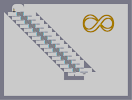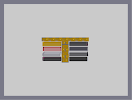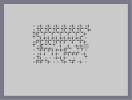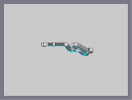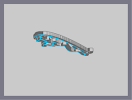Infinity Myspace Buttons Another, yet less frustrating, maze. Jag Test Jaguar Maserati Logo

Pages: (0)

### I'm patient...

and like to see what some pics look like when i do this.

But obviously this was just a message.

But most of the time, i can use photoshop to see how something would look.

I love getting comments on my work, and even though i dont submit anything, i still come every day to check on my maps.
Just to see if it has gotten any comments.

And a lot of the time, when the stuff is first posted, there is no need for returned comments. All OMG, or AMAZING at the bottom.
XD

### How did you do that :)

Can't wait to see your last thing.
I agree on the time you need to make those.
I almost never can be bothered to start working on one.
ahhhhhhh it's too... good my eyes!!!!

### wha...what the...@#\$% @#\$% @#\$% AAAAUUUUUUUUUUGGGHHHHHH!!!!!

HOW DID YOU DO THAT?!?!?!? YOU GOT TO TEACH ME!!!!! The only thing i know is the blue shade. nothing eltse.
That is the best text I've ever seen in a map.

### Oh yeah,

and have a good vacation.

typo

### Omg

how you do thes things is just incredible, but this is spot on. How you got the writing so perfect I will never know. Faved

Thats amazing :P

:)